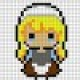# Binary Search Tree

picture credits :http://turnoff.us/image/en/binary-tree.png

So we have binary tree , but it was not sorted in a way . So difference between a binary tree ,

So we follow the nomenclature used below ,

Our class will look like,

1.Create:

Same as Binary Tree , except we don’t give user the choice to select position in the tree. Instead , we put value regarding to respect to it’s root value.

```void BST::Create()
{

Node *newnode;
int num;
int k=0;
do
{
cout<<"\n Enter the data :";
cin>>num;
newnode = new Node(num);
newnode->left=newnode->right=NULL;
if (root==NULL)
{
root=newnode;//It was empty
}
else{

temp=root;
while (1)
{
if(num<temp->data){
if (temp->left==NULL)
{
temp->left=newnode;
break;
}
else
temp=temp->left;
}
else
{
if (temp->right==NULL)
{
temp->right=newnode;
break;
}
else
temp=temp->right;
}

}

}
cout<<"To continue press 0 , Else press anything";
cin>>k;

} while (k==0);
}
```

2.Insert:

Similar to Create method ,

```void BST::Insert(int number){
Node*newnode;
newnode=new Node(number);
if (root==NULL)
root=newnode;
else
{
temp=root;
while(1)
{
if(number<temp->data){
if (temp->left==NULL)
{
temp->left=newnode;
break;
}
else
temp=temp->left;
}
else{
if (temp->right==NULL)
{
temp->right=newnode;
break;
}
else
temp=temp->right;
}
}
}
}
```

3.Search

```Node* BST::Search(Node * root,int val){

if(root!=NULL)
{
if(root->data ==val)
return root;
if(val<root->data)
return Search(root->left,val);
if(val>root->data)
return Search(root->right,val);
}
return NULL;
}
```

4.Delete

1. A leaf Node
2. A Node with one child
3. A Node with two child
``` Node* BST::del( Node *root ,int data) {

if (root == NULL) {
return NULL;
}
if (data < root->data) {  // data is in the left sub tree.
root->left = del(root->left,data);
}
else if (data > root->data) { // data is in the right sub tree.
root->right = del(root->right, data);
} else {
// case 1: no children
if (root->left == NULL && root->right == NULL) {
delete(root); // wipe out the memory, in C, use free function
root = NULL;
}
// case 2: one child (right)
else if (root->left == NULL) {
Node *temp = root; // save current node as a backup
root = root->right;
delete temp;
}
// case 3: one child (left)
else if (root->right == NULL) {
Node *temp = root; // save current node as a backup
root = root->left;
delete temp;
}
// case 4: two children
else {
Node *temp = FindMin(root->right); // find minimal value of right sub tree
root->data = temp->data; // duplicate the node
root->right = del(root->right, temp->data); // delete the duplicate node
}
}
return root; // parent node can update reference
}
```

5.Level Wise Display

```bool BST :: printLevel(Node* root, int level)
{
if (root == nullptr)
return false;

if (level == 1)
{
cout << root->data<< " ";

// return true if at-least one node is present at given level
return true;
}

bool left = printLevel(root->left, level - 1);
bool right = printLevel(root->right, level - 1);

return left || right;
}

void BST ::levelOrderTraversal(Node* root)
{
// start from level 1 -- till height of the tree
int level = 1;

// run till printLevel() returns false
while (printLevel(root, level))
level++;
}

```

### AVL TREE

It is named after its creator (Georgy Adelson-Velsky and Landis’ tree). In AVL Tree, the heights of child sub trees at any node differ by at most 1. At anytime if height difference becomes greater than 1 then tree balancing is done to restore its property.

It is a self balancing tree, now as it is self balanced and does not have the case of skewed trees , hence the time complexity of this Tree is always O(log n).

Now to utilize further more memory , we use leaf nodes. Where Leaf nodes where pointing to NULL, which would have been a waste. So to save memory , we pointed them in a particular order , such as in order ,pre order or post order.

*The leftmost and right most leaf node pointer , point towards a new Node called as Head or Dummy Node .

left _thread and right_thread =1 means they are pointing towards ancestors , whereas =0 means they are pointing towards child’s

Consider the below example , in order to understand the create function

1.Create Function

1. We check value of root , if root==NULL . It mean’s tree is empty till now.
2. Then we put value of new node into root , also make it point towards head or dummy node.Step 1
3. Then we assign temp=newnode , for iteration purposes
4. We then check value of number input with temp data
5. if it is less , it goes to left sub tree,where we check the value of left_thread to see if it is zero or not.
6. If zero then we newnode->left=temp->left;temp=temp=temp->left;temp->left_thread =1;
7. If it is more , then we check for right_thread to see if it is zero or not\
8. If zero then we newnode->right=temp->right;temp=temp=temp->right;temp->right_thread =1;
```void TBT::Create()
{

Node *newnode;
int num;
int k=0;
do
{
cout<<"\n Enter the data :";
cin>>num;
newnode = new Node(num);
if (root==NULL)
{
root=newnode;//It was empty
newnode->left=newnode->right=head;//to initialize the root pointing towards the head pointer , first step

}
else{

temp=newnode;
while (1)
{
if(num<temp->data){
{
newnode->left=temp->left;//Step 2 where the root->left == NULL is now pointing towards head
temp->left=newnode;
break;
}
else
temp=temp->left;
}
else
{
{
newnode->right=temp->right;//Step 3 where the root->left == NULL is now pointing towards head
temp->right=newnode;
break;
}
else
temp=temp->right;
}

}

}
cout<<"To continue press 0 , Else press anything";
cin>>k;

} while (k==0);
}
```

Binary tree in previous blog , https://programmerprodigy.code.blog/2020/05/02/trees-to-binary-trees/

GitHub code :https://github.com/kakabisht/Data-structures

1.tanvi pathak says: